Geometry

# Properties of Triangles: Level 2 Challenges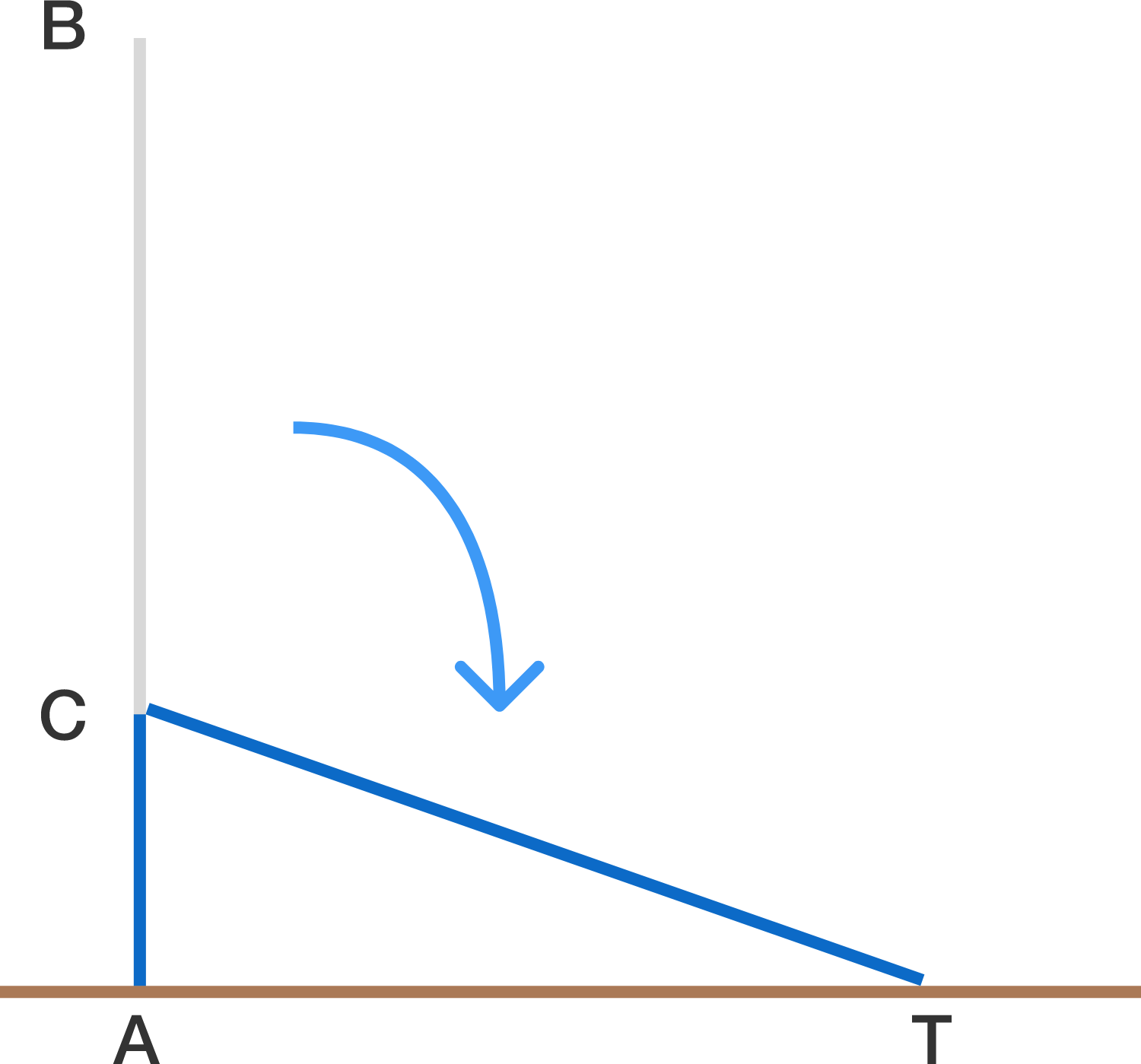A vertical pole AB measuring 5 meters snaps at point C. The pole remains in contact at C and the top of the pole touches the ground at point T, a distance of 3 meters from A.
Find the length AC, in meters, the point where the pole snapped.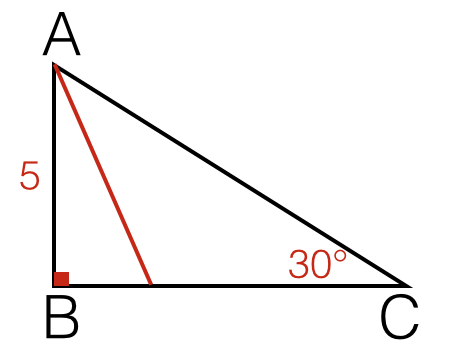Let $$ABC$$ be a triangle with $$\angle B=90^\circ$$, $$\angle C=30^\circ$$ and $$AB=5$$ Find the length of the angle bisector from $$A$$ to $$\overline{BC}$$(right to $$2$$ decimal places).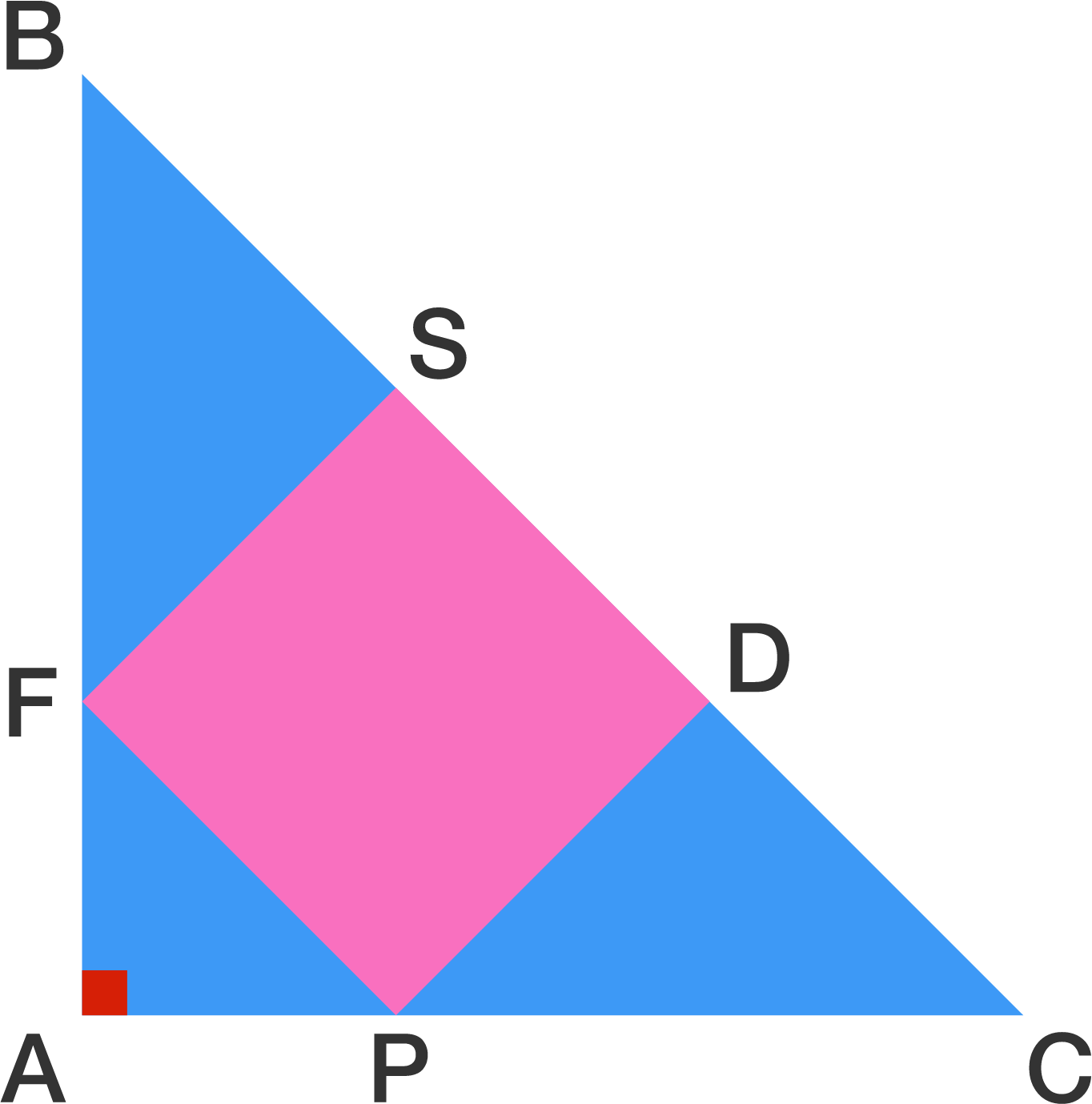$$ABC$$ is an isosceles right triangle with $$AB = AC = 6$$.

Given that $$SDPF$$ is a square, find the area of the square.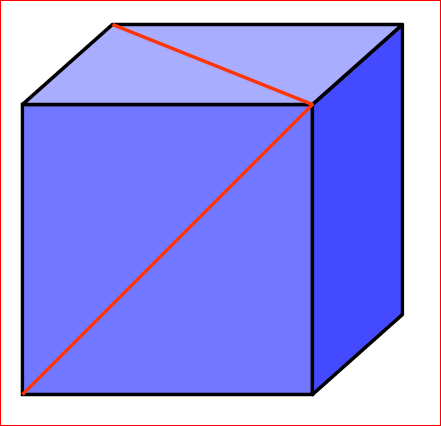The two red lines in the diagram shown are drawn diagonally on the faces of a cube. What is the angle (in degrees) between them at the point where they join?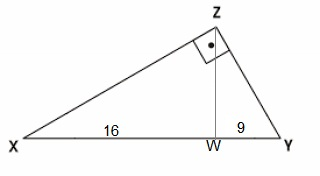In the image above, $$\overline { ZW } \bot \overline { XY },$$ $$\overline { XW } =16$$ and $$\overline { WY } =9$$. Find $$\overline { ZY } +\overline {XY }$$

×